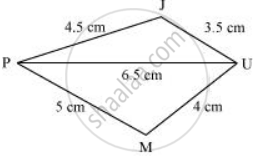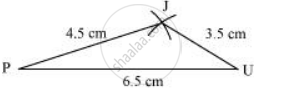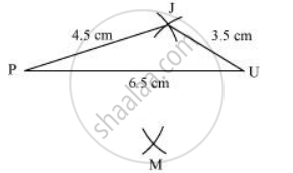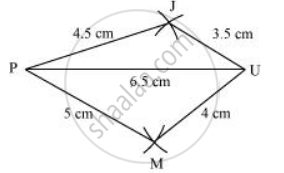Share

# Construct the Following Quadrilaterals. Quadrilateral Jump Ju = 3.5 Cm Um = 4 Cm Mp = 5 Cm Pj = 4.5 Cm Pu = 6.5 Cm - Mathematics

Course
ConceptConstructing a Quadrilateral When the Lengths of Four Sides and a Diagonal Are Given

JU = 3.5 cm

UM = 4 cm

MP = 5 cm

PJ = 4.5 cm

PU = 6.5 cm

#### Solution

Firstly, a rough sketch of this quadrilateral can be drawn as follows1) Δ JUP can be constructed by using the given measurements as follows2) Vertex M is 5 cm away from vertex P and 4 cm away from vertex U. Taking P and U as centres, draw arcs of radii 5 cm and 4 cm respectively. Let the point of intersection be M.3) Join M to P and U.